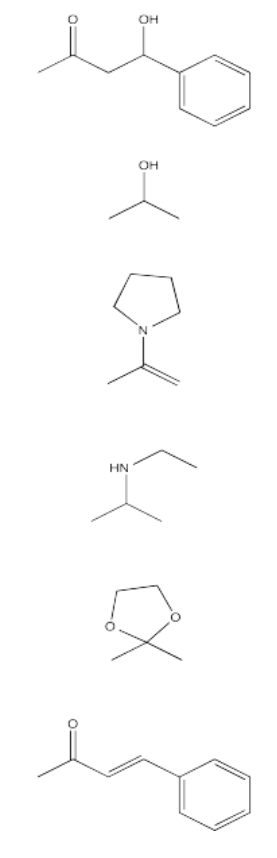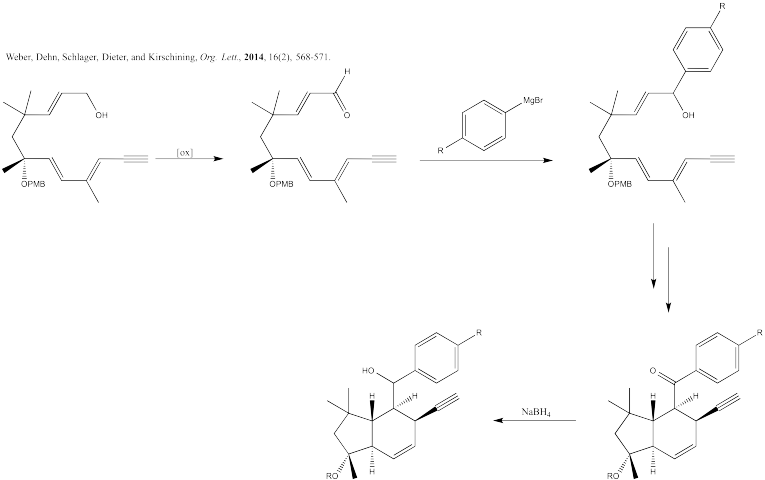Exercise $$\PageIndex{1}$$

Synthesize the following compounds starting from acetone.Exercise $$\PageIndex{2}$$

Researchers are investigating cyclohexenone derivatives as potential inhibitors for esterases. Below is a scheme for the synthesis of several of these derivatives. Fill in the boxes with the appropriate intermediates or reagents.Below is another derivative that they made. Provide a mechanism (make sure to draw arrows) for the following reaction:Exercise $$\PageIndex{3}$$

Fill in the product or reagent for each of the following transformations. Remember there is always an acidic workup assumed.Exercise $$\PageIndex{4}$$

Fill in the blanks in the following synthesis. Includes addition to carbonyls (anionic nucleophiles).Exercise $$\PageIndex{5}$$

Fill in the blanks in the following synthesis. Includes addition of nucleophiles to carbonyls (anionic nucleophiles, enolates, ylides).Exercise $$\PageIndex{6}$$

Fill in the blanks in the following synthesis. Includes addition of nucleophiles to carbonyls (anionic nucleophiles, enolates).Exercise $$\PageIndex{7}$$

Fill in the blanks in the following synthesis. Includes addition of nucleophiles to carbonyls (anionic nucleophiles, enolates, ylides).Exercise $$\PageIndex{8}$$

Fill in the blanks in the following synthesis. Includes addition of nucleophiles to carbonyls (anionic nucleophiles, enolates, conjugate additions, carboxyloid substitutions).Exercise $$\PageIndex{9}$$

Fill in the blanks in the following synthesis. Includes addition of nucleophiles to carbonyls (anionic nucleophiles, enolates, conjugate addition).